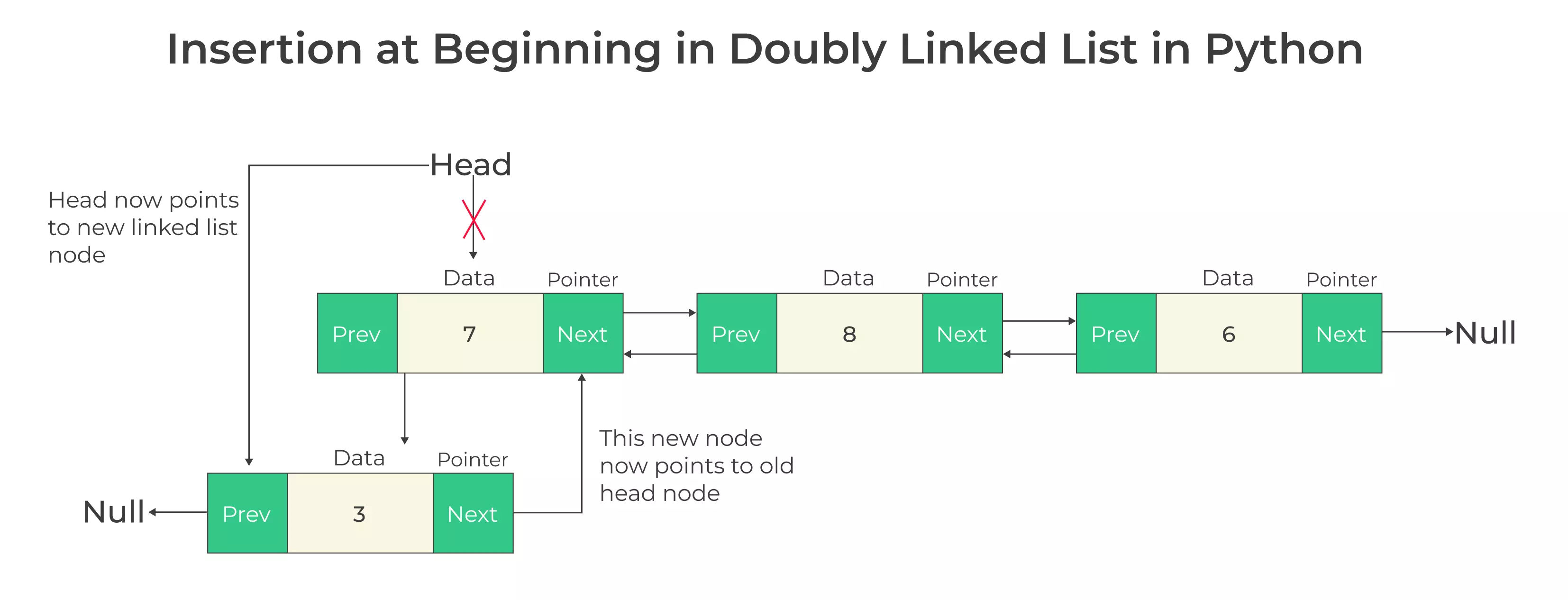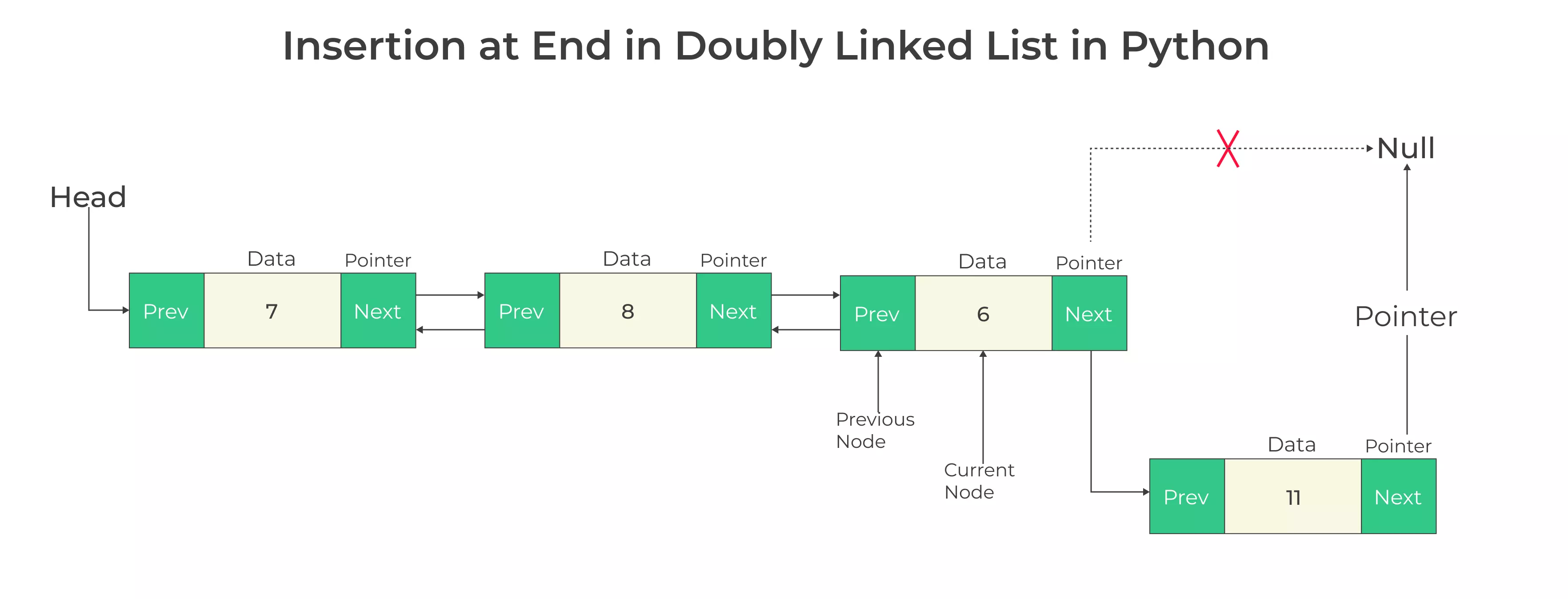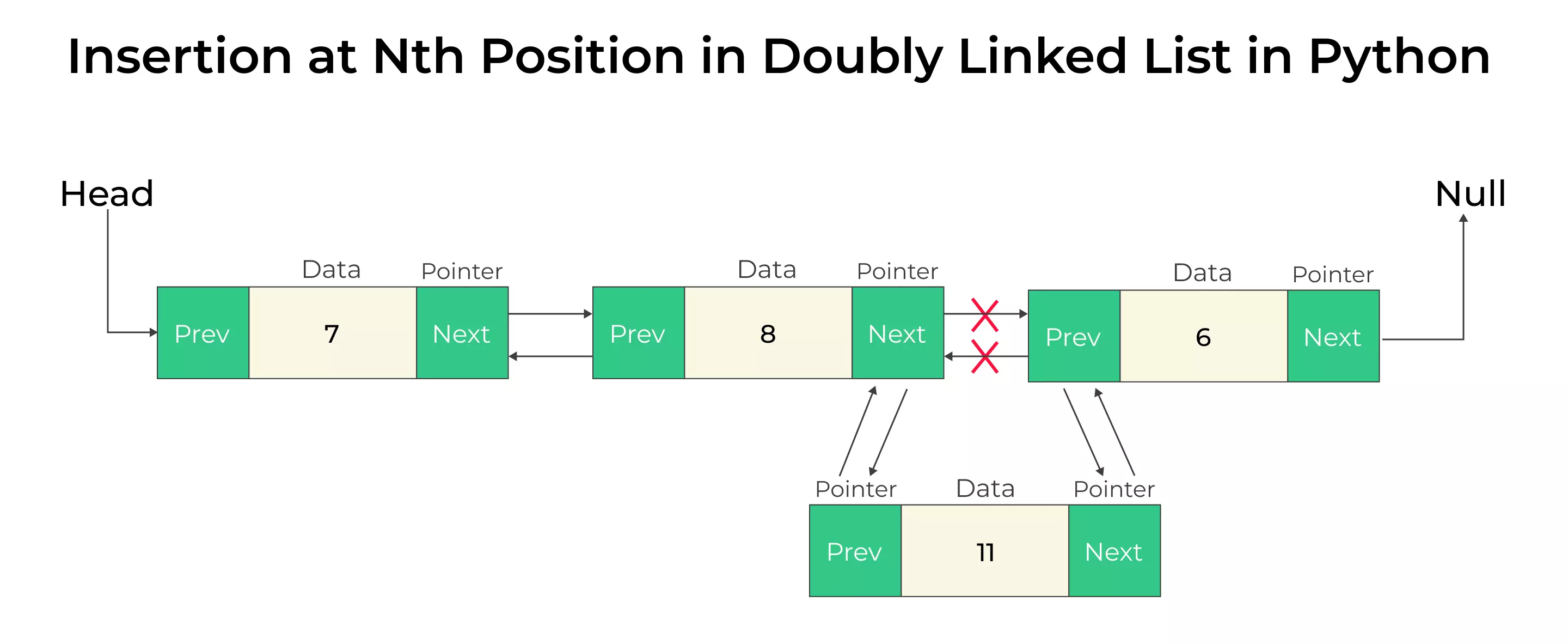# Insertion in Doubly Linked List in Python

## Insertion in Doubly Linked List in Python

Doubly linked lists are a fundamental data structure in computer science and are often used in various applications to efficiently manage and manipulate data.

In this page, we will explore how to perform insertion in doubly linked list using Python. We will cover different scenarios and techniques for inserting elements at various positions within the list## Understanding Doubly Linked Lists in Python

A Doubly Linked List is a data structure that consists of a set of nodes, where each node contains both data and two pointers: one pointing to the next node and another pointing to the previous node. This bidirectional linking enables efficient traversal in both directions.

### Elements of Doubly Linked List

• Data : This is the actual information or value that the node holds. It can be of any data type, depending on the application’s requirements. For example, in a list of student records, the data may include the student’s name, ID, and grade.

• Next Pointer : The “next” pointer, also known as the “forward” or “next node” pointer, points to the next node in the list. It helps in navigating from the current node to the next node when traversing the list in the forward direction.

• Previous Pointer : The “previous” pointer, also known as the “backward” or “previous node” pointer, points to the previous node in the list. It facilitates traversal in the reverse direction, allowing you to move from the current node to the previous node.

For each insertion operation, we need to consider the three cases. These three cases also need to be considered when removing data from the doubly linked list.

• Insertion at the beginning
• Insertion at end
• Insertion at Nth position

```# Define a Node class to represent a doubly linked list node
class Node:
def __init__(self, data):
self.data = data
self.next = None
self.prev = None
```### Insertion at Beginning in Doubly Linked List in Python

• Create a new node with the desired data.

• Update the pointers of the new node to point to the current first node and NULL for the previous node.

• Update the previous pointer of the current first node to point to the new node.

• Update the head pointer to point to the new node, making it the new first node.

```# Function to create a new node
def createNode(data):
new_node = Node(data)  # Assuming you have the Node class defined as shown earlier
return new_node

# Create a new node
newNode = createNode(data)

# Update pointers
newNode.prev = None

# Update the previous pointer of the current first node

```

### Python Code(Insertion at Beginning)

```class Node:
def __init__(self, data):
self.data = data
self.next = None
self.prev = None

def __init__(self):

def insert_at_beginning(self, data):
new_node = Node(data)
```

### Insertion at End in Doubly Linked List in Python

• Create a new node with the desired data.

• Traverse the list to find the last node.

• Update the pointers of the new node to point to the current last node as the previous node and NULL as the next node.

• Update the next pointer of the current last node to point to the new node.

```# Create a new node
newNode = createNode(data)

# Traverse to find the last node
while current.next is not None:
current = current.next

# Update pointers
newNode.prev = current
newNode.next = None
current.next = newNode
```### Python Code(Insertion at End)

```class DoublyLinkedList:
# ... (previous code)

def insert_at_end(self, data):
new_node = Node(data)
return
while current.next:
current = current.next
current.next = new_node
new_node.prev = current

```

### Insertion at Nth Position in  Doubly Linked List in Python

• Calculate the size of the node
• If the position you want to enter is less than 1
• Invalid position But, if 0 then use insertAtStart method
• If the position you want to enter is greater than size then
• Invalid position, but if the position is equal to size then use insertLast method
• else create a temp node and assign it the address of the head
• Create a new node and assign it the data value Iterate to the position you want to enter after in the linked list
• Assign this new node’s next and previous nodes
• Assign previous node’s next to this new node
• Assign next node’s previous to this new node### Python Code(Insertion at Nth Position)

```class Node:
def __init__(self, data):
self.data = data
self.next = None
self.prev = None

new_node = Node(data)
new_node.next = None

new_node.prev = None
return

while temp.next is not None:
temp = temp.next

temp.next = new_node
new_node.prev = temp

new_node = Node(data)
new_node.prev = None

def calcSize(node):
size = 0

while node is not None:
node = node.next
size += 1

return size

if pos < 0 or pos > size:
print("Can't insert, {} is not a valid position".format(pos))
return

if pos == 0:
elif pos == size:
else:
new_node = Node(data)
new_node.next = None

while pos > 0:
temp = temp.next
pos -= 1

temp2 = temp.next
new_node.next = temp2
new_node.prev = temp
temp.next = new_node
temp2.prev = new_node

def display(node):
end = None
print("List in Forward direction:", end=" ")
while node is not None:
print(node.data, end=" ")
end = node
node = node.next
print("\nList in backward direction:", end=" ")
while end is not None:
print(end.data, end=" ")
end = end.prev

# Main function
if __name__ == "__main__":
print("Linked list before insertion at specific position")
print("\n\nLinked list after insertion at specific position")
```

#### Output

```linked before insertion at specific position
List in Forward direction:  20  16  12  10  14  18  11
List in backward direction:  11  18  14  10  12  16  20

linked after insertion at specific position
List in Forward direction:  20  16  12  25  10  14  18  11
List in backward direction:  11  18  14  10  25  12  16  20```

#### Singly vs Doubly Linked List Insertion in python

Data StructureEach node contains data and a reference to the next node.Each node contains data, a reference to the next node, and a reference to the previous node.
Insertion at the BeginningO(1)O(1)
Insertion at the EndO(n) – Requires traversing the entire list to find the last node.O(1) – Can directly access the last node.
Insertion at a Specific PositionO(n) – Requires traversing the list to find the desired position.O(n) – Requires traversing the list to find the desired position.
Insertion after a NodeO(1) if you have a reference to the node, O(n) if you need to find the node first.O(1) if you have a reference to the node, O(n) if you need to find the node first.
Deletion EfficiencyO(n) – Requires traversing to find and remove the node.O(1) – Can directly remove a node if you have a reference to it.
Traversal EfficiencyO(n) – Requires traversing the list from the beginning.O(n) – Can traverse forward and backward.
Use CasesSuitable when primarily dealing with forward traversal. Memory-efficient for simple scenarios.Suitable when frequent forward and backward traversal is needed. Offers more flexibility but with higher memory overhead.

#### Conclusion

In this page, we have discussed about insertion in doubly linked list in Python and concept of doubly linked lists and how to perform various insertion operations in python. We’ve covered inserting at the beginning, inserting at the end, and inserting at Nth position. These operations are crucial when working with doubly linked lists and can be adapted to suit your specific needs in different programming scenarios.

### Related Banners

Get PrepInsta Prime & get Access to all 200+ courses offered by PrepInsta in One SubscriptionQuestion 1.

What is a doubly linked list?

A doubly linked list is a data structure in which each node contains two pointers: one pointing to the previous node and another pointing to the next node.Question 2.

Doubly linked lists allow for bidirectional traversal, making certain operations more efficient compared to singly linked lists.Question 3.

How do I delete a node from a doubly linked list?

To delete a node, you can update the previous node’s next’ pointer to skip the node you want to delete and update the next node’s prev’ pointer accordingly.

## Get over 200+ course One Subscription

Courses like AI/ML, Cloud Computing, Ethical Hacking, C, C++, Java, Python, DSA (All Languages), Competitive Coding (All Languages), TCS, Infosys, Wipro, Amazon, DBMS, SQL and others